SNOSAZ6C August   2008  – November 2015

PRODUCTION DATA.

1. Features
2. Applications
3. Description
1.     Device Images
4. Revision History
5. Pin Configuration and Functions
6. Specifications
7. Detailed Description
1. 7.1 Overview
2. 7.2 Functional Block Diagram
3. 7.3 Feature Description
1. 7.3.1 Input Characteristics
2. 7.3.2 EMIRR
3. 7.3.3 EMIRR Definition
4. 7.4 Device Functional Modes
8. Application and Implementation
1. 8.1 Application Information
2. 8.2 Typical Application
9. Power Supply Recommendations
10. 10Layout
11. 11Device and Documentation Support
12. 12Mechanical, Packaging, and Orderable Information

• DCK|5
• DCK|5

6.7 Typical Characteristics

At TA = 25°C, RL = 10 kΩ, V+ = 3.3 V, V = 0 V, Unless otherwise specified.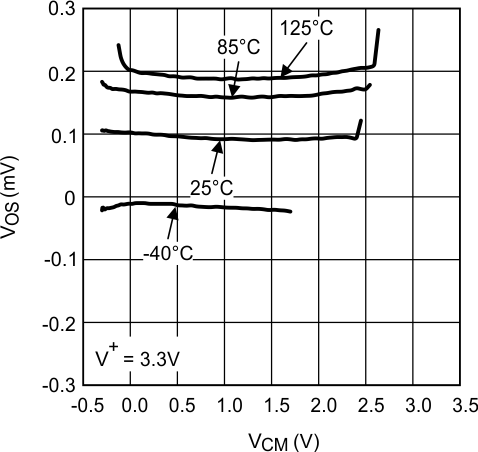Figure 1. VOS vs VCM at V+ = 3.3 V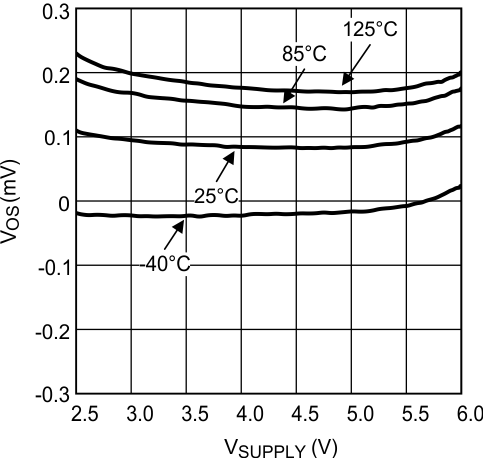Figure 3. VOS vs Supply Voltage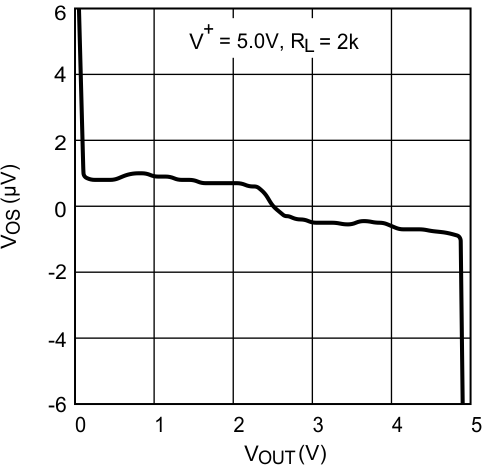Figure 5. VOS vs VOUT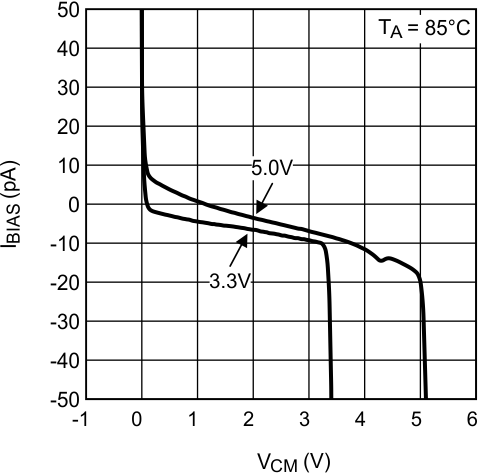Figure 7. Input Bias Current vs VCM at 85°C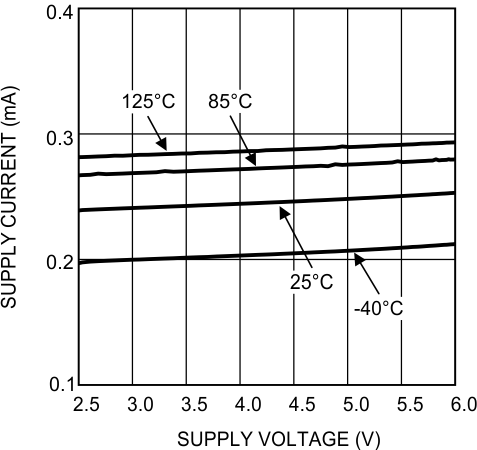Figure 9. Supply Current vs Supply Voltage Single LMV831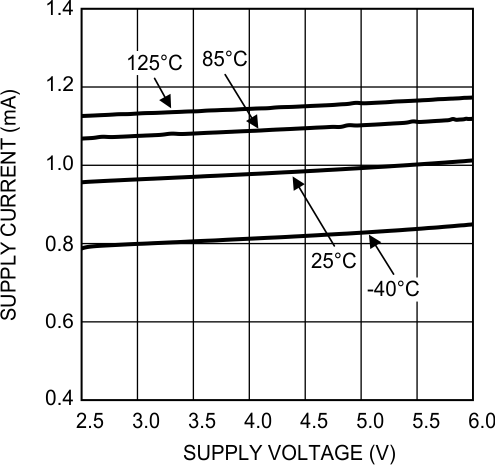Figure 11. Supply Current vs Supply Voltage Quad LMV834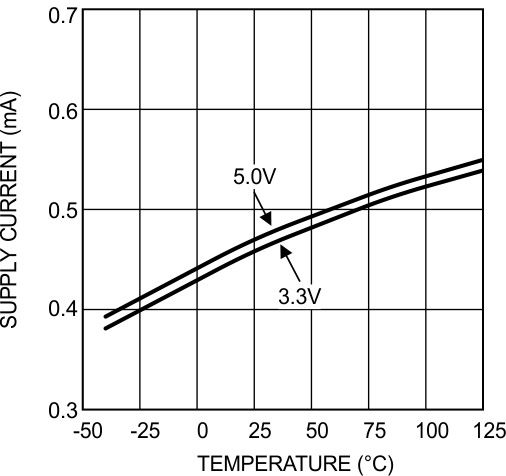Figure 13. Supply Current vs Temperature Dual LMV832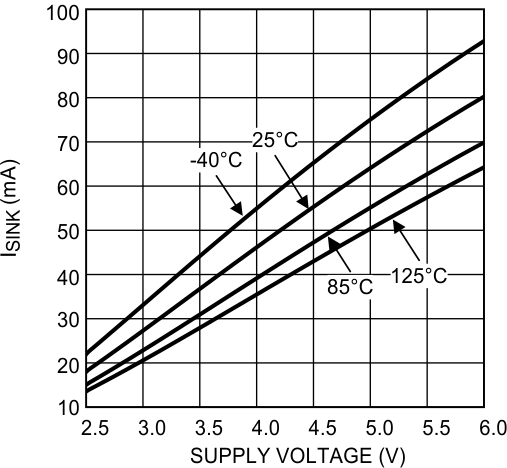Figure 15. Sinking Current vs Supply Voltage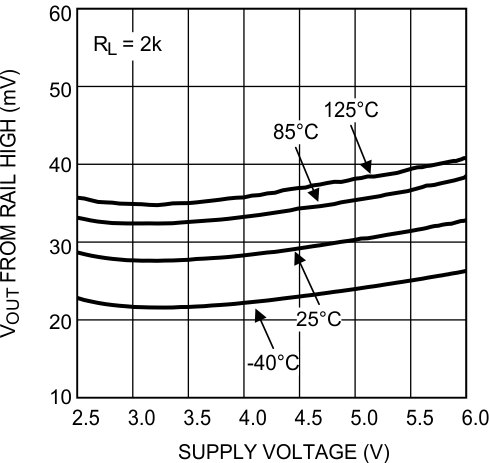RL = 2 kΩ
Figure 17. Output Swing High vs Supply Voltage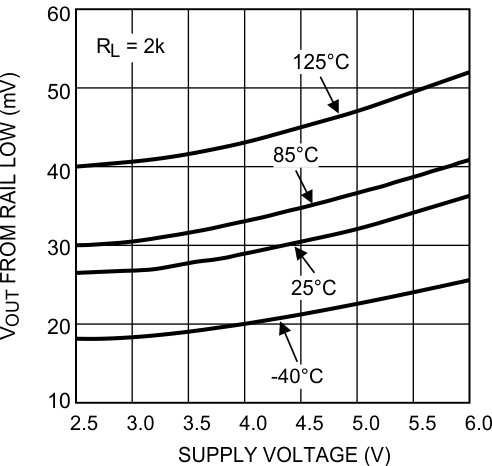RL = 2 kΩ
Figure 19. Output Swing Low vs Supply Voltage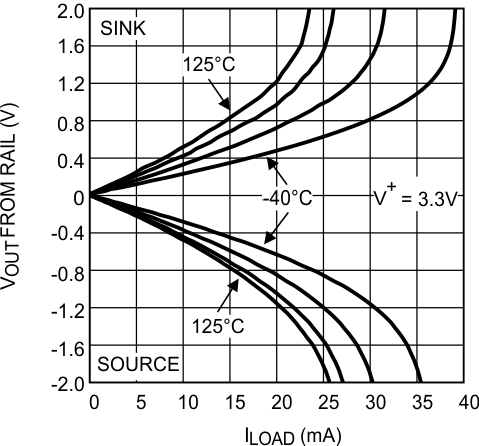Figure 21. Output Voltage Swing vs Load Current at V+ = 3.3 V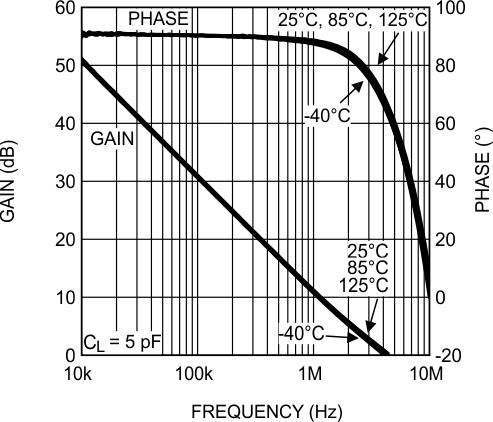Figure 23. Open-Loop Frequency Response vs Temperature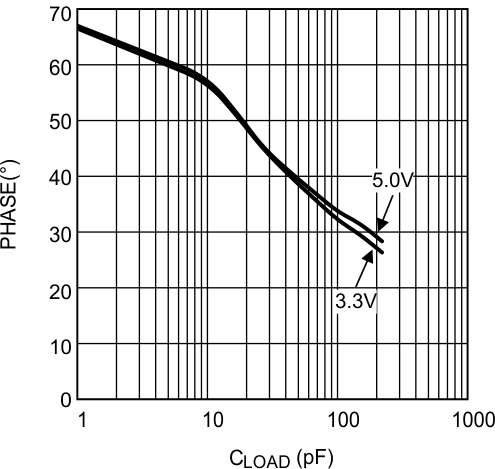Figure 25. Phase Margin vs Capacitive Load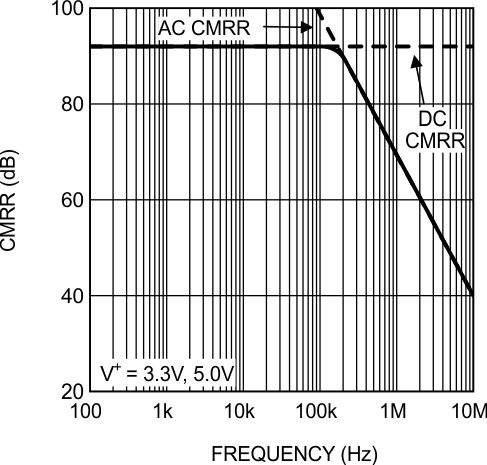Figure 27. CMRR vs Frequency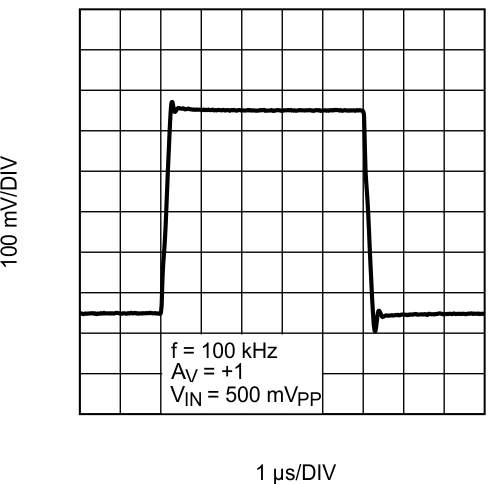Figure 29. Large Signal Step Response With Gain = 1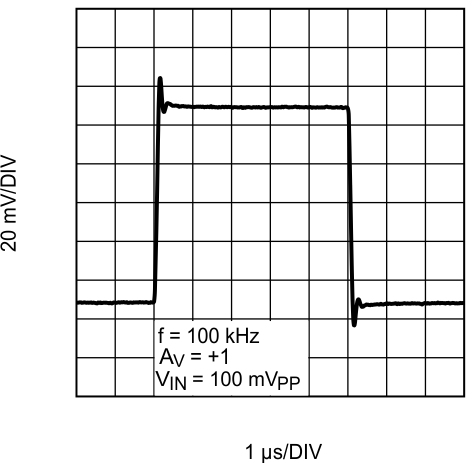Figure 31. Small Signal Step Response With Gain = 1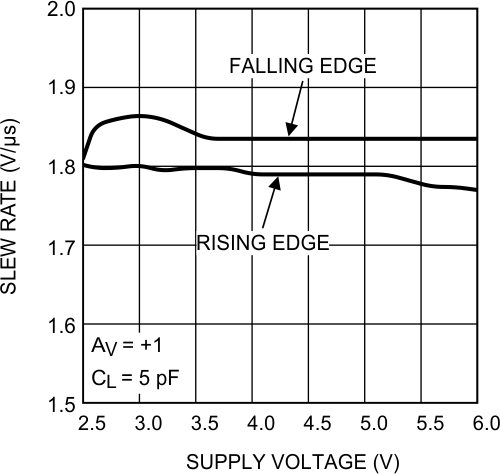Figure 33. Slew Rate vs Supply Voltage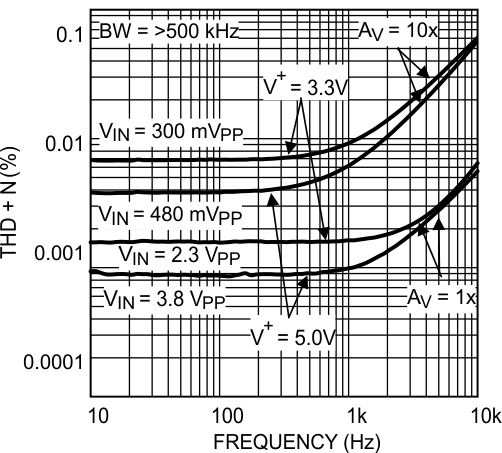Figure 35. THD+N vs Frequency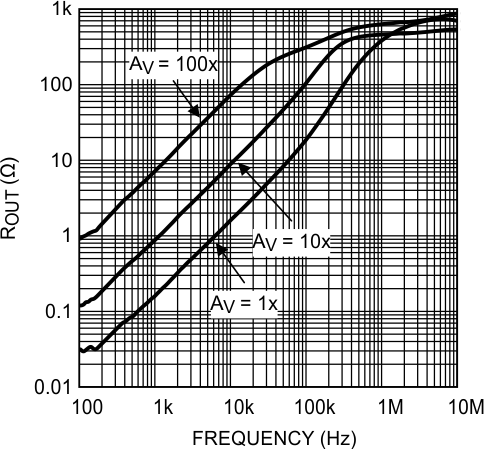Figure 37. ROUT vs FrequencyFigure 39. EMIRR IN+ vs Power at 900 MHz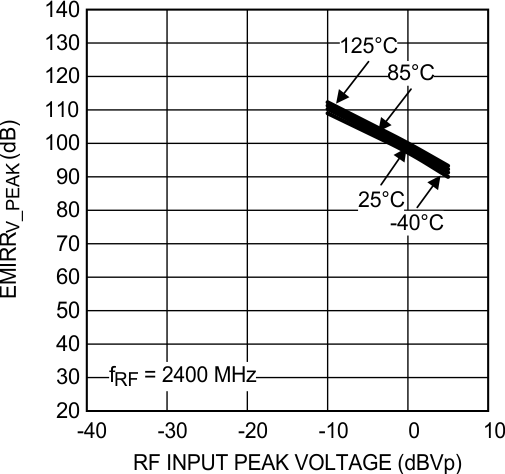Figure 41. EMIRR IN+ vs Power at 2400 MHz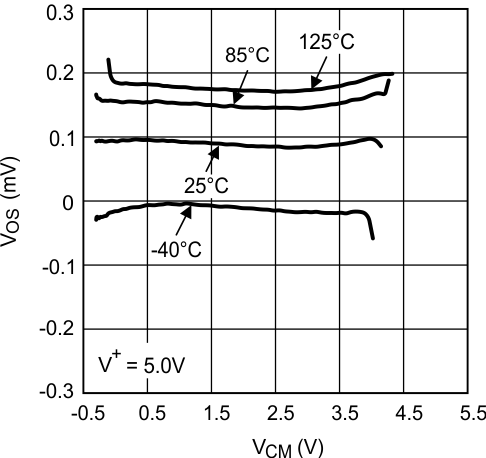Figure 2. VOS vs VCM at V+ = 5 V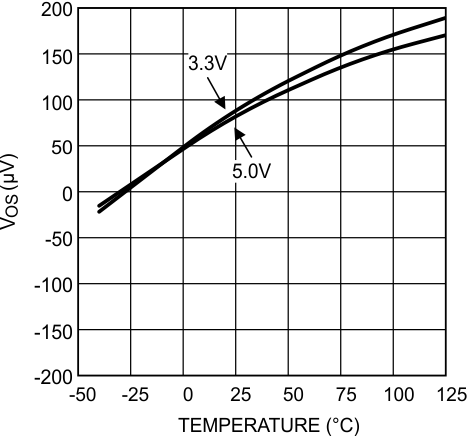Figure 4. VOS vs Temperature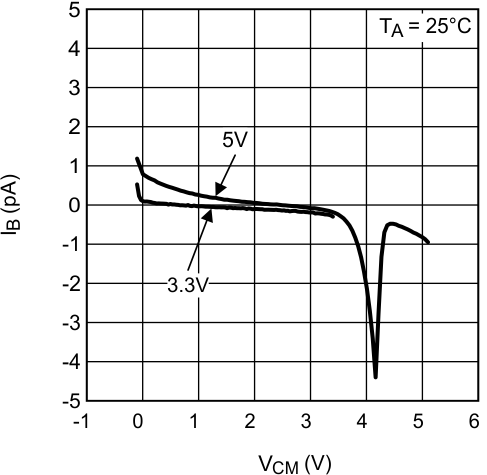Figure 6. Input Bias Current vs VCM at 25°CFigure 8. Input Bias Current vs VCM at 125°C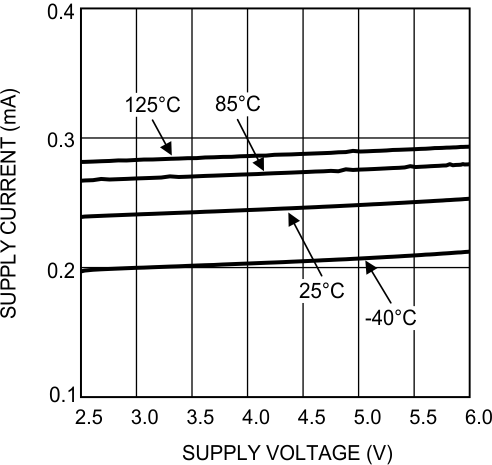Figure 10. Supply Current vs Supply Voltage Dual LMV832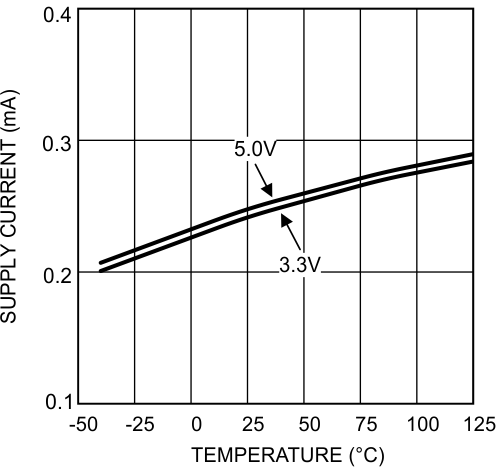Figure 12. Supply Current vs Temperature Single LMV831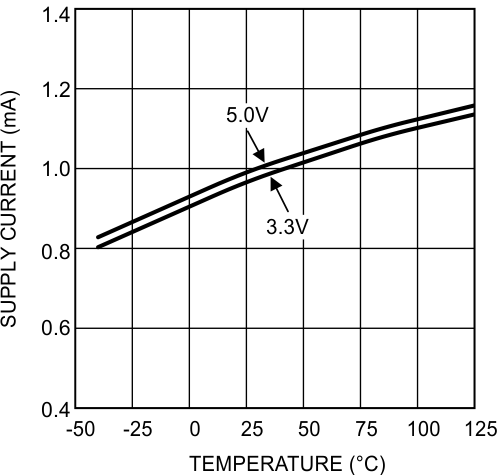Figure 14. Supply Current vs Temperature Quad LMV834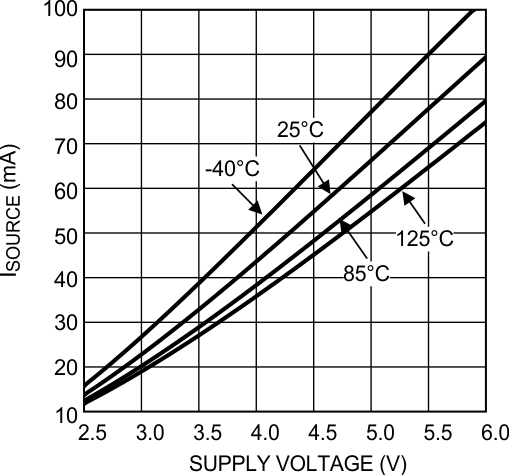Figure 16. Sourcing Current vs Supply Voltage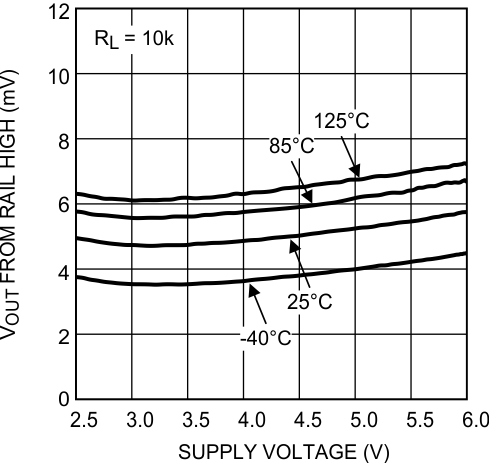RL = 10 kΩ
Figure 18. Output Swing High vs Supply Voltage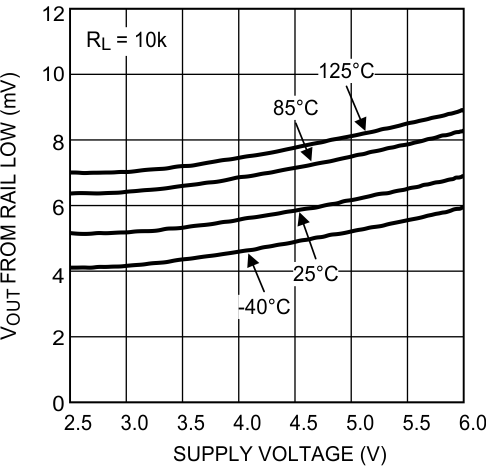RL = 10 kΩ
Figure 20. Output Swing Low vs Supply Voltage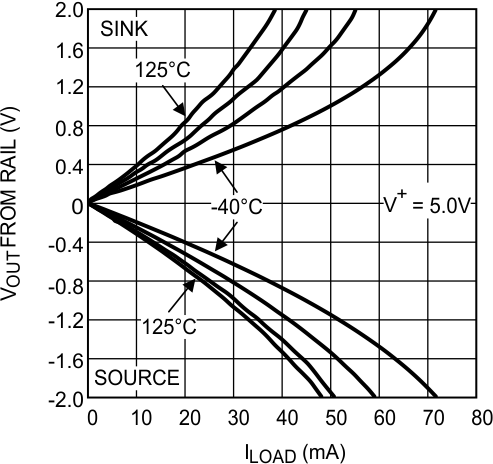Figure 22. Output Voltage Swing vs Load Current at V+ = 5 V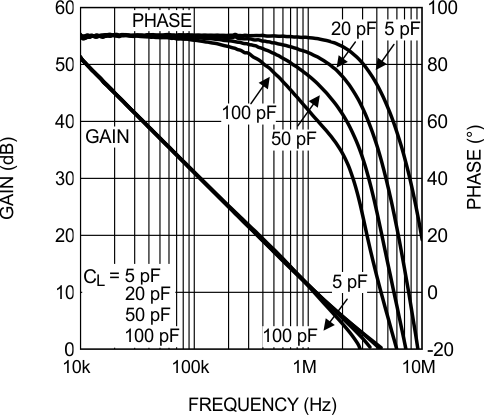Figure 24. Open-Loop Frequency Response vs Load Conditions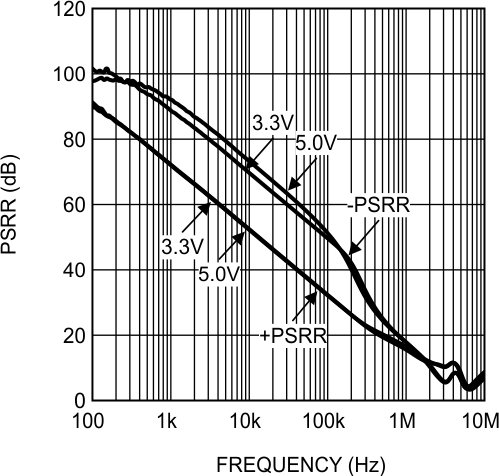Figure 26. PSRR vs Frequency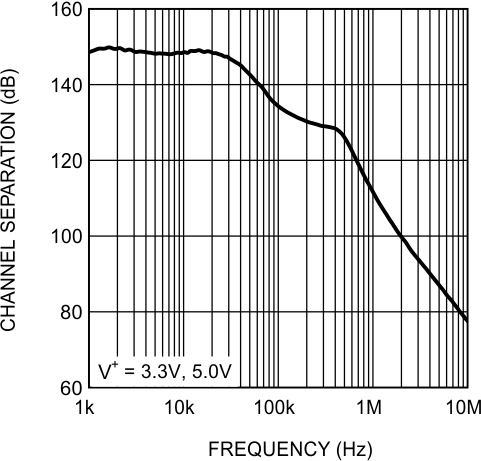Figure 28. Channel Separation vs Frequency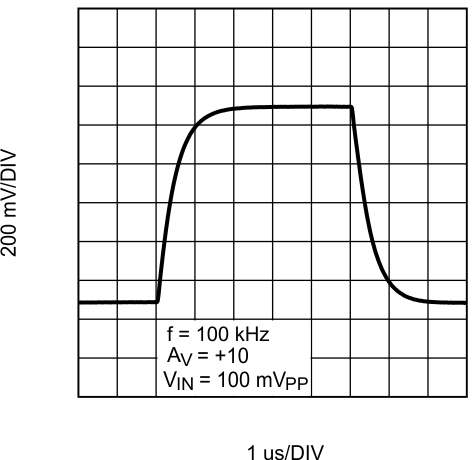Figure 30. Large Signal Step Response With Gain = 10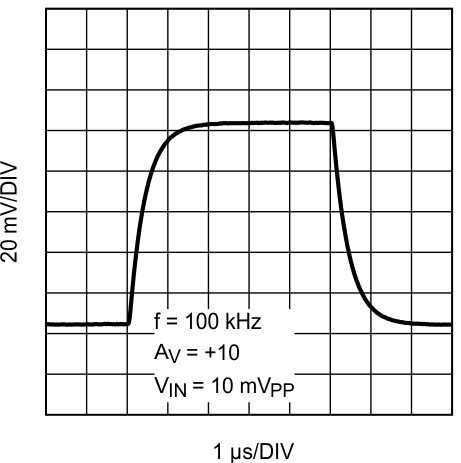Figure 32. Small Signal Step Response With Gain = 10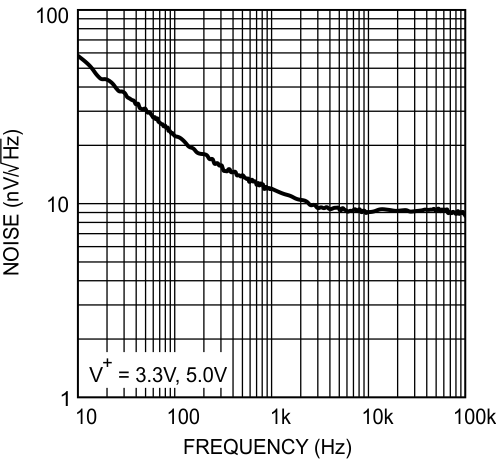Figure 34. Input Voltage Noise vs Frequency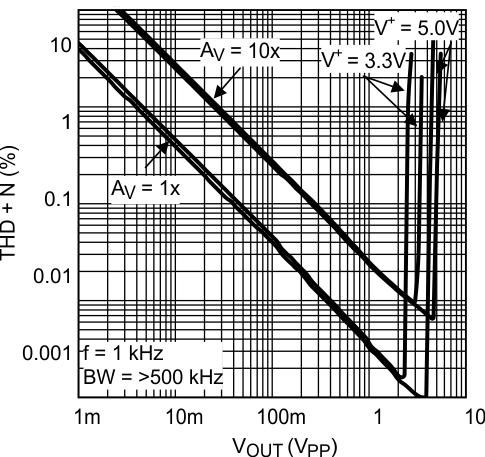Figure 36. THD+N vs AmplitudeFigure 38. EMIRR IN+ vs Power at 400 MHz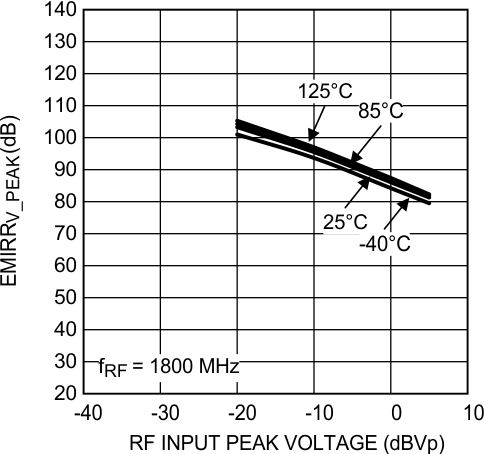Figure 40. EMIRR IN+ vs Power at 1800 MHz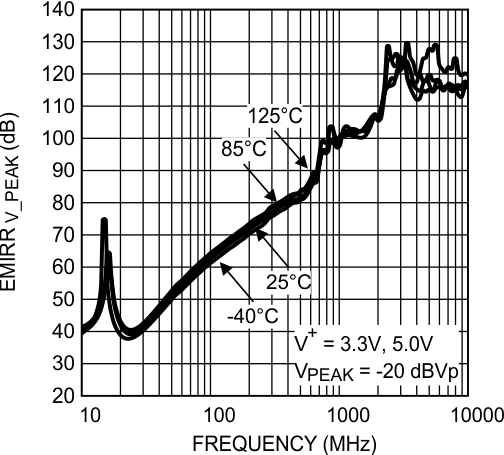Figure 42. EMIRR IN+ vs Frequency